# Basic Arithmetic : Changing a Percent to a Decimal

## Example Questions

### Example Question #1 : Changing View

Change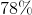to a decimal.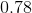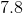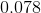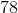Explanation:

To change a percent to a decimal, drop the "%" sign then divide by 100.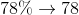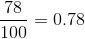### Example Question #31 : Percents And Decimals

What is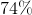as a decimal?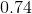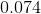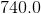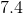Explanation:

To change a percent by the decimal, first drop the percent sign.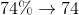Then, divide the number by 100.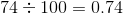### Example Question #31 : Percents And Decimals

Convert the following percent to a decimal: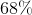.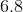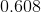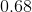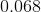Explanation:

To convert a percent into a decimal, divide the percent by 100 or move the decimal point 2 places to the left.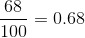or 68 hundredths.

### Example Question #101 : Basic Arithmetic

Convert the following percent to a decimal: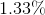.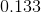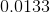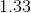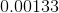Explanation:

To convert a percent to a decimal, divide the percent by 100 or move the decimal point two places to the left.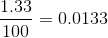### Example Question #31 : Percents And Decimals

One out of the three shirts I own is red. What percent of my shirts are not red? Round your final answer to the nearest tenth.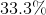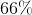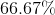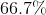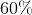Explanation:

The question is in regards to the amount of non-red shirts. If one of the three shirts is red, then two are not red. We represent this as a fraction:In order to convert it into a percent, we carry out the division and multiply the result by 100: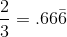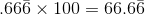We want to round to the nearest tenth, which is the number directly to the right of the decimal point. The number in the next spot over, the hundredths place, is six, so we round the tenths spot up to seven. This gives us a final answer of 66.7%.

### Example Question #32 : Percents And Decimals

What is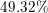expressed as a decimal?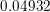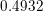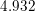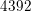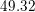Explanation:

Percentages are numbers represented as parts of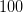therefore meansparts of. To get the corresponding decimal, we need to divide by(which can be done by moving the decimal point two places to the left) and remove the percentage sign. Thus we obtain: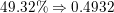Our final answer is.

### All Basic Arithmetic Resources